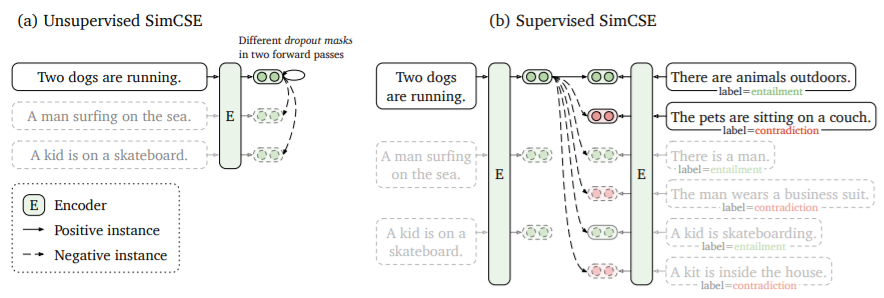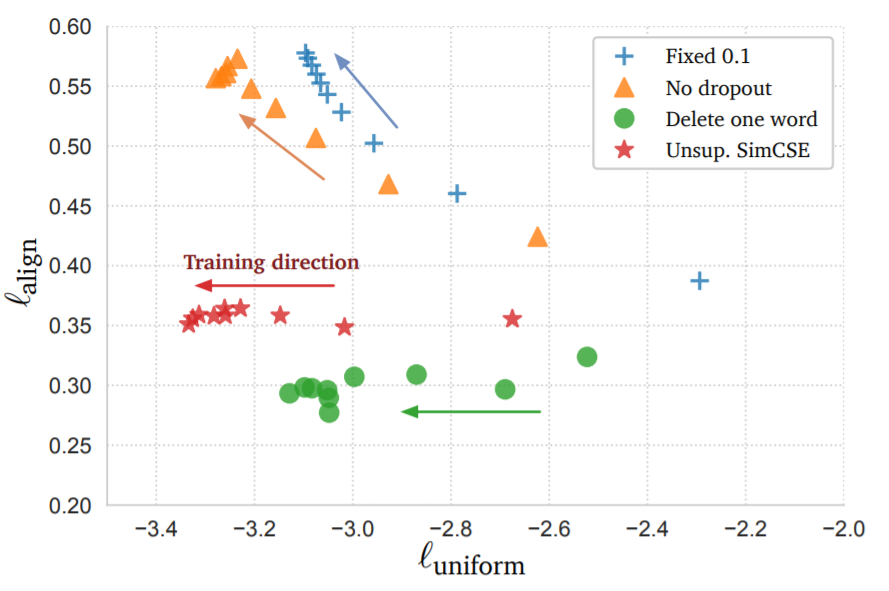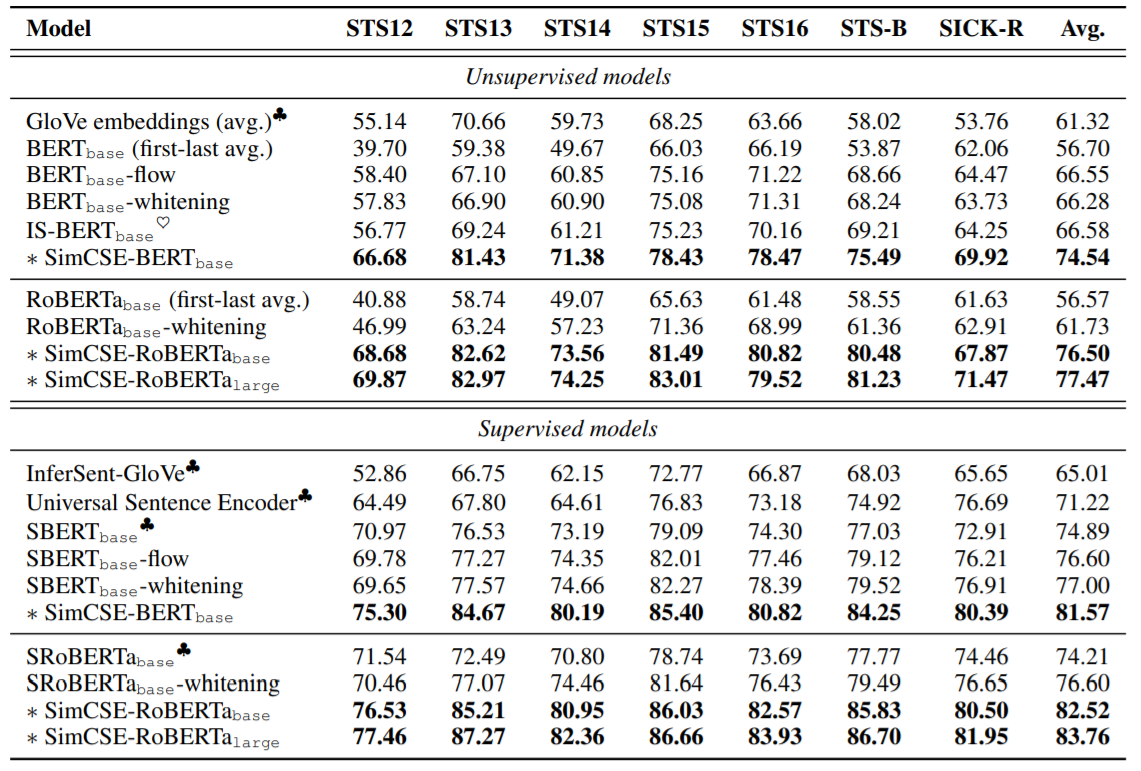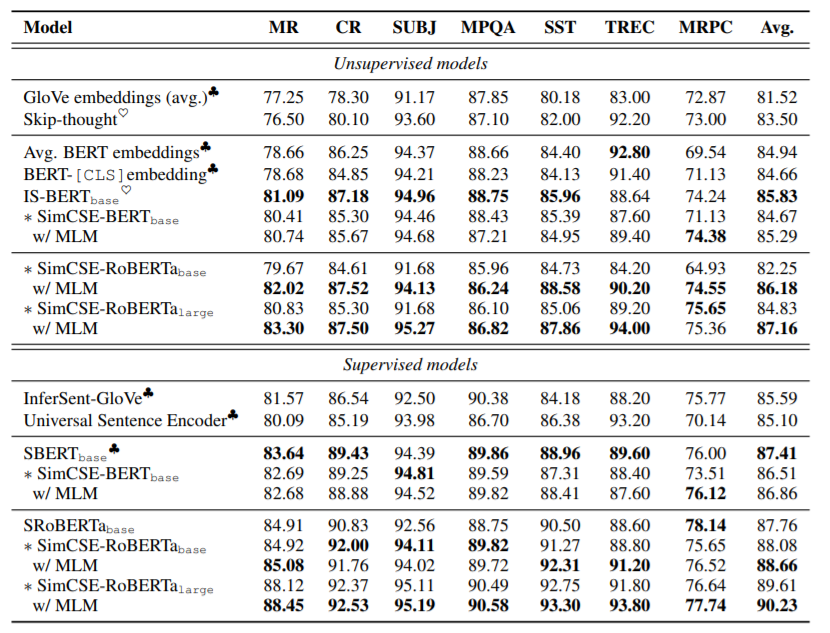## Overview

• Arxiv: https://arxiv.org/pdf/2104.08821v1.pdf
• Code: https://github.com/princeton-nlp/SimCSE

## Background: Contrastive Learning

### Positive instances

align描述了样本对之间的距离 l_{\rm align} \triangleq \mathop{\mathbb{E}}_{(x,x^+)\sim p_{pos}}\|f(x)-f(x^+)\|^2 uniform描述了样本是否分布均匀 $l_{\rm uniform} \triangleq \log\mathop{\mathbb{E}}_{(x,y)\sim p_{data}}e^{-2\|f(x)-f(y)\|^2}$## Experiment